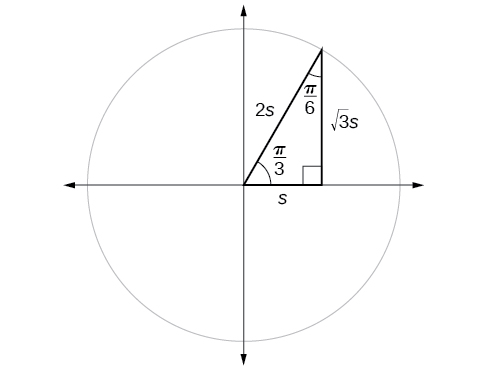7.2 Right triangle trigonometry  (Page 3/12)

 Page 3 / 12

Find the exact value of the trigonometric functions of $\text{\hspace{0.17em}}\frac{\pi }{4},$ using side lengths.

$\begin{array}{ccc}\hfill \text{sin}\left(\frac{\theta }{4}\right)& =& \frac{\sqrt{2}}{2},\text{cos}\left(\frac{\pi }{4}\right)=\frac{\sqrt{2}}{2},\mathrm{tan}\left(\frac{\pi }{4}\right)=1,\hfill \\ \hfill \text{sec}\left(\frac{\pi }{4}\right)& =& \sqrt{2},\text{csc}\left(\frac{\pi }{4}\right)=\sqrt{2},\text{cot}\left(\frac{\pi }{4}\right)=1\hfill \end{array}$

Using equal cofunction of complements

If we look more closely at the relationship between the sine and cosine of the special angles, we notice a pattern. In a right triangle with angles of $\text{\hspace{0.17em}}\frac{\pi }{6}\text{\hspace{0.17em}}$ and $\text{\hspace{0.17em}}\frac{\pi }{3},$ we see that the sine of $\text{\hspace{0.17em}}\frac{\pi }{3},$ namely $\text{\hspace{0.17em}}\frac{\sqrt{3}}{2},$ is also the cosine of $\text{\hspace{0.17em}}\frac{\pi }{6},$ while the sine of $\text{\hspace{0.17em}}\frac{\pi }{6},$ namely $\text{\hspace{0.17em}}\frac{1}{2},$ is also the cosine of $\text{\hspace{0.17em}}\frac{\pi }{3}.$

$\begin{array}{cccc}\hfill \mathrm{sin}\frac{\pi }{3}& =\mathrm{cos}\frac{\pi }{6}\hfill & =\frac{\sqrt{3}s}{2s}\hfill & =\frac{\sqrt{3}}{2}\hfill \\ \hfill \mathrm{sin}\frac{\pi }{6}& =\mathrm{cos}\frac{\pi }{3}\hfill & =\frac{s}{2s}\hfill & =\frac{1}{2}\hfill \end{array}$The sine of   π 3   equals the cosine of   π 6   and vice versa.

This result should not be surprising because, as we see from [link] , the side opposite the angle of $\text{\hspace{0.17em}}\frac{\pi }{3}\text{\hspace{0.17em}}$ is also the side adjacent to $\text{\hspace{0.17em}}\frac{\pi }{6},$ so $\text{\hspace{0.17em}}\mathrm{sin}\left(\frac{\pi }{3}\right)\text{\hspace{0.17em}}$ and $\text{\hspace{0.17em}}\mathrm{cos}\left(\frac{\pi }{6}\right)\text{\hspace{0.17em}}$ are exactly the same ratio of the same two sides, $\text{\hspace{0.17em}}\sqrt{3}s\text{\hspace{0.17em}}$ and $\text{\hspace{0.17em}}2s.\text{\hspace{0.17em}}$ Similarly, $\text{\hspace{0.17em}}\mathrm{cos}\left(\frac{\pi }{3}\right)\text{\hspace{0.17em}}$ and $\text{\hspace{0.17em}}\mathrm{sin}\left(\frac{\pi }{6}\right)\text{\hspace{0.17em}}$ are also the same ratio using the same two sides, $\text{\hspace{0.17em}}s\text{\hspace{0.17em}}$ and $\text{\hspace{0.17em}}2s.$

The interrelationship between the sines and cosines of $\text{\hspace{0.17em}}\frac{\pi }{6}\text{\hspace{0.17em}}$ and $\text{\hspace{0.17em}}\frac{\pi }{3}\text{\hspace{0.17em}}$ also holds for the two acute angles in any right triangle, since in every case, the ratio of the same two sides would constitute the sine of one angle and the cosine of the other. Since the three angles of a triangle add to $\text{\hspace{0.17em}}\pi ,$ and the right angle is $\text{\hspace{0.17em}}\frac{\pi }{2},$ the remaining two angles must also add up to $\text{\hspace{0.17em}}\frac{\pi }{2}.\text{\hspace{0.17em}}$ That means that a right triangle can be formed with any two angles that add to $\text{\hspace{0.17em}}\frac{\pi }{2}\text{\hspace{0.17em}}$ —in other words, any two complementary angles. So we may state a cofunction identity : If any two angles are complementary, the sine of one is the cosine of the other, and vice versa. This identity is illustrated in [link] .

Using this identity, we can state without calculating, for instance, that the sine of $\text{\hspace{0.17em}}\frac{\pi }{12}\text{\hspace{0.17em}}$ equals the cosine of $\text{\hspace{0.17em}}\frac{5\pi }{12},$ and that the sine of $\text{\hspace{0.17em}}\frac{5\pi }{12}\text{\hspace{0.17em}}$ equals the cosine of $\text{\hspace{0.17em}}\frac{\pi }{12}.\text{\hspace{0.17em}}$ We can also state that if, for a given angle then $\text{\hspace{0.17em}}\mathrm{sin}\left(\frac{\pi }{2}-t\right)=\frac{5}{13}\text{\hspace{0.17em}}$ as well.

Cofunction identities

 $\mathrm{cos}\text{\hspace{0.17em}}t=\mathrm{sin}\left(\frac{\pi }{2}-t\right)$ $\mathrm{sin}\text{\hspace{0.17em}}t=\mathrm{cos}\left(\frac{\pi }{2}-t\right)$ $\mathrm{tan}\text{\hspace{0.17em}}t=\mathrm{cot}\left(\frac{\pi }{2}-t\right)$ $\mathrm{cot}\text{\hspace{0.17em}}t=\mathrm{tan}\left(\frac{\pi }{2}-t\right)$ $\mathrm{sec}\text{\hspace{0.17em}}t=\mathrm{csc}\left(\frac{\pi }{2}-t\right)$ $\mathrm{csc}\text{\hspace{0.17em}}t=\mathrm{sec}\left(\frac{\pi }{2}-t\right)$

Given the sine and cosine of an angle, find the sine or cosine of its complement.

1. To find the sine of the complementary angle, find the cosine of the original angle.
2. To find the cosine of the complementary angle, find the sine of the original angle.

Using cofunction identities

If $\text{\hspace{0.17em}}\mathrm{sin}\text{\hspace{0.17em}}t=\frac{5}{12},$ find $\text{\hspace{0.17em}}\mathrm{cos}\left(\frac{\pi }{2}-t\right).$

According to the cofunction identities for sine and cosine, we have the following.

$\mathrm{sin}\text{\hspace{0.17em}}t=\mathrm{cos}\text{\hspace{0.17em}}\left(\frac{\pi }{2}-t\right)$

So

$\mathrm{cos}\text{\hspace{0.17em}}\left(\frac{\pi }{2}-t\right)=\frac{5}{12}$

If $\text{\hspace{0.17em}}\mathrm{csc}\text{\hspace{0.17em}}\left(\frac{\pi }{6}\right)=2,$ find $\text{\hspace{0.17em}}\mathrm{sec}\text{\hspace{0.17em}}\left(\frac{\pi }{3}\right).$

2

Using trigonometric functions

In previous examples, we evaluated the sine and cosine in triangles where we knew all three sides. But the real power of right-triangle trigonometry emerges when we look at triangles in which we know an angle but do not know all the sides.

Given a right triangle, the length of one side, and the measure of one acute angle, find the remaining sides.

1. For each side, select the trigonometric function that has the unknown side as either the numerator or the denominator. The known side will in turn be the denominator or the numerator.
2. Write an equation setting the function value of the known angle equal to the ratio of the corresponding sides.
3. Using the value of the trigonometric function and the known side length, solve for the missing side length.

A laser rangefinder is locked on a comet approaching Earth. The distance g(x), in kilometers, of the comet after x days, for x in the interval 0 to 30 days, is given by g(x)=250,000csc(π30x). Graph g(x) on the interval [0, 35]. Evaluate g(5)  and interpret the information. What is the minimum distance between the comet and Earth? When does this occur? To which constant in the equation does this correspond? Find and discuss the meaning of any vertical asymptotes.
The sequence is {1,-1,1-1.....} has
how can we solve this problem
Sin(A+B) = sinBcosA+cosBsinA
Prove it
Eseka
Eseka
hi
Joel
June needs 45 gallons of punch. 2 different coolers. Bigger cooler is 5 times as large as smaller cooler. How many gallons in each cooler?
7.5 and 37.5
Nando
find the sum of 28th term of the AP 3+10+17+---------
I think you should say "28 terms" instead of "28th term"
Vedant
the 28th term is 175
Nando
192
Kenneth
if sequence sn is a such that sn>0 for all n and lim sn=0than prove that lim (s1 s2............ sn) ke hole power n =n
write down the polynomial function with root 1/3,2,-3 with solution
if A and B are subspaces of V prove that (A+B)/B=A/(A-B)
write down the value of each of the following in surd form a)cos(-65°) b)sin(-180°)c)tan(225°)d)tan(135°)
Prove that (sinA/1-cosA - 1-cosA/sinA) (cosA/1-sinA - 1-sinA/cosA) = 4
what is the answer to dividing negative index
In a triangle ABC prove that. (b+c)cosA+(c+a)cosB+(a+b)cisC=a+b+c.
give me the waec 2019 questionsByByByByBy